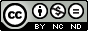Please use this identifier to cite or link to this item: `https://scidar.kg.ac.rs/handle/123456789/8844`
 Title: Relationship between the indexes of insulin resistance and metabolic status in dairy cows during early lactation Authors: Cincovic M.Kirovski D.Vujanac I.Belic B.Đoković, Radojica Issue Date: 2017 Abstract: © 2017, Walter de Gruyter GmbH. All rights reserved. Insulin resistance is a phenomenon which accompanies the ongoing metabolic adaptation in cows during early lactation. The aim of our study was to determine the linear correlations of HOMA (Homeostatic Model Assessment), QUICKI (Quantitative Insulin Sensitivity Check Index) and RQUICKI (Revised Quantitative Insulin Sensitivity Check Index) indexes of insulin resistance with the metabolic status of cows (concentration of hormones, metabolites and body condition score). The experiment included 40 Holstein-Frisian cows in the first week after calving. Indexes of insulin resistance valued: 18.68±5.43 (HOMA), 0.39±0.06 (QUICKI) and 0.45±0.06 (RQUICKI). Linear correlations were examined by testing the coefficient of correlation (r), determination (r2,%) and regression parameter beta (b) in linear equation. A negative correlation was found between HOMA and IGF-I (insulin growth factor I) (r=-0.51, r2=25.0, b=-1.1257, p<0.01). HOMA showed a positive correlation with BHB (beta-hidroxybutyrate) (r=0.48, r2=23.2, b=0.0234, p<0.01). A positive correlation was found between QUICKI and IGF-I (r=0.30, r2=10.0 b=46.7900, p<0.05) and cholesterol (r=0.44, r2=18.3, b=1.9021, p<0.01). In contrast, QUICKI and BHB (r=0.51, r2=27.1, b=-1.7241, p<0.01), just like QUICKI and BCS (r=0.46, r2=20.9, b=-2.424, p<0.01), showed a negative correlation. RQUICKI showed positive correlations with IGF-I (r=0.48, r2=22.8, b=28.1230, p<0.01), T4 (r=0.47, r2=22.1, b=87.142, p<0.01) and triglycerides (r=0.36, r2=13, b=0.0407, p<0.05) but negative correlations with cortisol (r=-0.36, r2=13.0, b=-9.0332, p<0.05), STH (somatotropic hormone) (r=-0.42, r2=17.3, b=-5.4976, p<0.01), BHB (r=-0.62, r2=38.3, b=-1.1872, p<0.01), total bilirubin (r=-0.58, r2=33.7, b=-7.131, p<0.01) and BCS (body condition score) (r=-0.6, r2=36.4, b=-1.8347, p<0.01). In conclusion, indexes of insulin resistance may be used to evaluate the metabolic status of cows in early lactation. RQUICKI might be the most appropriate predictor of metabolic status due to its linear relationship with most of the parameters included in homeorhetic process. URI: https://scidar.kg.ac.rs/handle/123456789/8844 Type: article DOI: 10.1515/acve-2017-0006 ISSN: 0567-8315 SCOPUS: 2-s2.0-85016710637 Appears in Collections: Faculty of Agronomy, Čačak#### Page views(s)

64

This item is licensed under a Creative Commons License